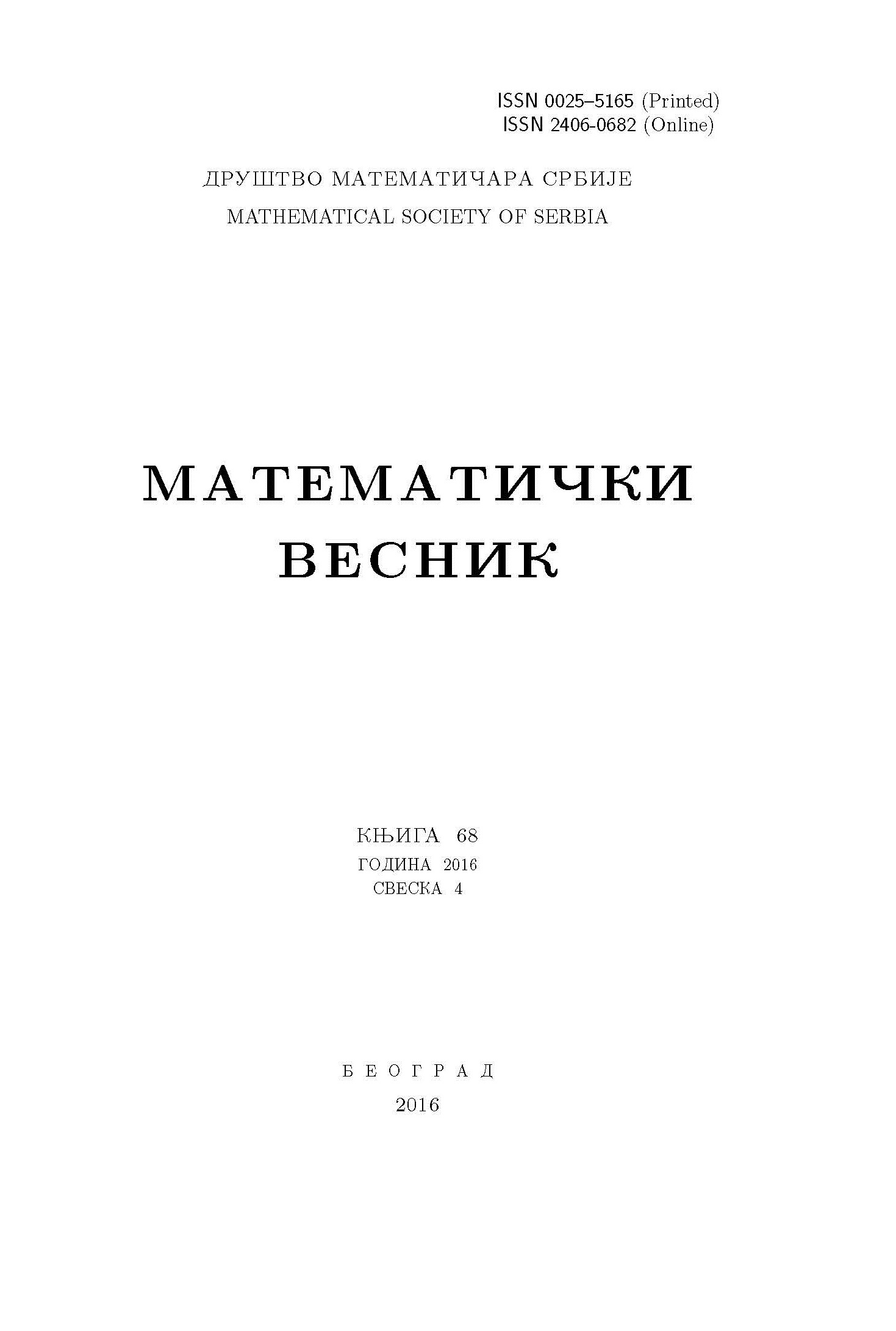﻿ Matematički Vesnik ﻿
 MATEMATIČKI VESNIK МАТЕМАТИЧКИ ВЕСНИКSOME RESULTS ON SCALABLE $K$-FRAMES S. Ramesan, K.T. Ravindran AbstractWe investigate the scalability of $K$-frames and derive a characterization for scalable $K$-frames. We investigate whether or not a particular $K$-frame is scalable, as well as the existence and uniqueness of scalings. Using the concept of trace of an operator, we analyse the possible scalings, if a given $K$-frame is scalable. In $\mathbb{C}^{n}$, we look at the scalability of $K$-frames independently.Keywords: Frames; $K$-frames; scalable $K$-frames. MSC: 42C15, 47A63 Pages:  1$-$10

﻿